# VR - Codes

Example 1

If the code for C R E A M is D S F B N then what is the code for B A N K S?

If you have been working your way through the letter series questions elsewhere, you should recognise the basic tenets of this question. The transposition of letters is what it's all about; crack the code of how many places are being moved and you'll probably be able to see the answer.

In this type of question, relate the first letter of the first word, ‘cream’, to the first letter of the second word, ‘dsfbn’. Next, look at the second letter of each. As you will find, this is a simple code where each of the code letters is one along in the alphabet from the original. In other words, C becomes D, R becomes S and so on. This is pretty straightforward - there won't be anything quite so easy in the real test!

Now we can work out the answer – B must become C, A becomes B, N becomes O, K becomes L and S becomes T. The code for BANKS is therefore CBOLT. Remember, the 'code' does not have to make sense as a word.

Example 2

If the code for B O O K is A Q N M then what is the code for T Y P E?

Let’s start by dealing with it letter by letter and applying the same rule for the first pair of words to the second.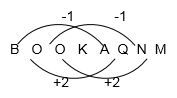As you can see, the pattern is not simple like it was in example 51. However, it does follow a regular pattern, albeit an alternating one. If we apply a similar rule for each letter of T Y P E then we get the following: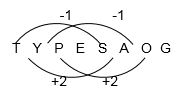Again, be aware that the alphabet in these questions is a loop – go one letter back from A and you get Z! The answer to the question is S A O G.

Don't forget that, as it is a 'code' that we are looking for, there is no need to be finding a legitimate 'word' as the answer. If there was a question working the other way round, and you had a code but needed to find a 'word', then you must check that it is a recognisable word!

Example 3

If the code for T I M E is S G J A then what is L Y F H the code for?

Let’s apply the same process as we did in the previous example but this time we need to do things in reverse to find the answer.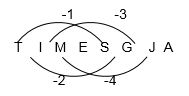The first code letter - S - is one back from T. The second is two back, then three, then four. In order to find the answer, we need to go from the new code back to the word it must represent. Therefore we have to go in reverse. Instead of reaching the code we need to reach the word that created it.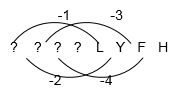To find the first letter, we must add one back on to the code letter ‘L’. The second letter is found by adding two back on to ‘Y’ (remembering that the alphabet is on a loop).

The answer will look like this: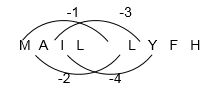Therefore the answer is M A I L.